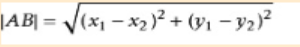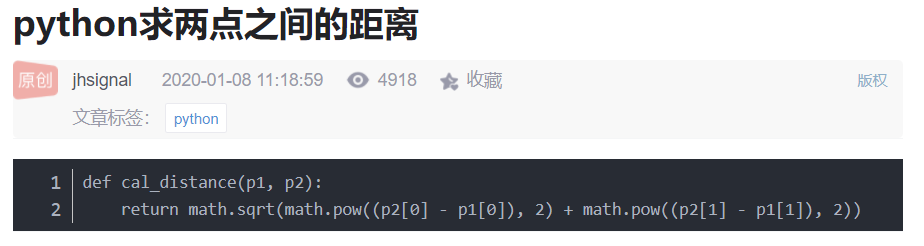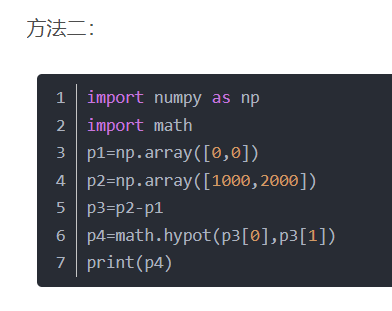• 2022-04-04 12:20:12

已知两个点的经度和纬度，计算两个点之间的距离

import pyproj

lat1,long1 = (37.8101,-122.4105)
lat2,long2 = (37.8024,-122.4058)

geod = pyproj.Geod(ellps="WGS84")

angle1,angle2, distance = geod.inv(long1,lat1,long2,lat2)

print(format(distance))


该例子来自《Python Geospatial Development》一书，此处仅作为学习笔记。

更多相关内容
• 如下所示： distances = np.sqrt(np.sum(np.asarray(airportPosition - x_vals)**2, axis=1)) ... 您可能感兴趣的文章:python计算两个地址之间的距离方法Python实现的计算马氏距离算法示例Python实现计
• 今天小编就为大家分享一篇python计算两个地址之间的距离方法，具有很好的参考价值，希望对大家有所帮助。一起跟随小编过来看看吧
• 计算两点之间的距离日常旁白：本人是一枚生物学的学生，由于对生物信息学特别感兴趣，于是想自学生物信息学（新手莫怪）。了解到生物信息学要有编程基础，尤其是要会一门编程语言，例如：R语言、Python、Perl等，...

计算出两点之间的距离

日常旁白：本人是一枚生物学的学生，由于对生物信息学特别感兴趣，于是想自学生物信息学（新手莫怪）。了解到生物信息学要有编程基础，尤其是要会一门编程语言，例如：R语言、Python、Perl等，还要熟悉Linux系统，作为生信小白，听说Python挺简单的，于是就自学了Python，花了两天时间了解了Python的基础语法后，今天想做个练习题试试手（实践是检验真理的唯一标准），下面是练习题：(试题来源：《PYTHON生物信息学数据管理》)试题02

书中答案原代码如下：

from math import *

x1,y1,z1 = 0.1,0.0,-0.7

x2,y2,z2 = 0.5,-1.0,2.7

dx = x1-x2

dy = y1-y2

dz = z1-z2

dsquare = pow(dx,2)+pow(dy,2)+pow(dz,2)

d = sqrt(dsquare)

print(d)

为了将所学内容活用，充分理解思路，特拆分讲解。（嫌麻烦的可以直接查看最后的升级版代码）

第一步：导入math模块

from math import *

第二步：获取两个点的坐标

①方便随时计算用户输入的两点的坐标并计算出两个点之间的距离。特增加了获取用户输入的input()函数。

#获取用户输入

x1,y1,z1=input("请依次输入点1纵横坐标的值：",)

x2,y2,z2=input("请依次输入点2纵横坐标的值：",)

②为了简化同时获取用户输入的点的坐标的三个值，使用了切片功能。split(',')表示以“，”作为分隔符，切割用户输入的三个字符。

x1,y1,z1=input("请依次输入点1纵横坐标的值：",).split(',')

x2,y2,z2=input("请依次输入点2纵横坐标的值：",).split(',')

第三步：编写计算公式

①计算差值

由于系统默认用户输入的是字符，所以使用下面的代码计算可能会报错

dx = x1-x2

dy = y1-y2

dz = z1-z2

因此增加了eval（）函数，将字符串转化为数值，方便下面的计算，代码如下：

dx = eval(x1) -eval(x2)

dy = eval(y1) -eval(y2)

dz = eval(z1) -eval(z2)

②计算公式

计算公式代码如下：

dsquare = pow(dx,2)+pow(dy,2)+pow(dz,2)

d = sqrt(dsquare)

第四步：输出结果

print（d）

最后将代码总结如下：

from math import *

#获取用户输入

x1,y1,z1=input("请依次输入点1纵横坐标的值：",).split(',')

x2,y2,z2=input("请依次输入点2纵横坐标的值：",).split(',')

dx = eval(x1) -eval(x2)

dy = eval(y1) -eval(y2)

dz = eval(z1) -eval(z2)

dsquare = pow(dx,2)+pow(dy,2)+pow(dz,2)

d = sqrt(dsquare)

print(d)

虽然这是个小小的计算程序，但对于初学者的我来说每一次对原代码的升级改造，哪怕是读懂后的注释都感觉是一次进步提升，总之代码虽小，动手最重要！希望更多学习Python的爱好者不要像我一样眼高手低，学习编程就是要，思考，敲码，思考，敲码，敲码，再敲码！

展开全文• 详细: 1.闵可夫斯基距离(Minkowski Distance) 2.欧氏距离(Euclidean Distance) ...两个n维变量A(x11,x12,…,x1n)与 B(x21,x22,…,x2n)间的闵可夫斯基距离定义为： 其中p是一个变参数。 当p=1时，就是曼哈顿距
• 计算两点(x1,y1),(x2,y2)之间的距离，输出并保留一位小数 两之间距离的数学公式： txt=input("请输入四数字，用空格隔开:") ls=txt.split(" ") x1=eval(ls) y1=eval(ls) x2=eval(ls) y2=eval(ls) r...

从键盘输入4个数字，采用空格分隔，对应变量为x1,y1,x2,y2。计算两点(x1,y1),(x2,y2)之间的距离，输出并保留一位小数

两点之间距离的数学公式：txt=input("请输入四个数字，用空格隔开:")
ls=txt.split(" ")
x1=eval(ls)
y1=eval(ls)
x2=eval(ls)
y2=eval(ls)
r=pow(pow(x1-x2,2)+pow(y1-y2,2),0.5)
print("{:.1f}".format(r))展开全文开发语言 后端
• #定义的函数 class Point: def __init__(self,x=0,y=0): self.x=x self.y=y def getx(self): return self.x def gety(self): return self.y #定义直线函数 class Getlen: def __init__(self,p1,p2): self...
• 算了，找了半天，就用第一种方法吧 dis = math.hypot(10,10) 计算和0,0之间的距离算了，找了半天，就用第一种方法吧

dis = math.hypot(10,10)

计算该点和0,0之间的距离

展开全文• 处理地理数据时，经常需要用到两个地理位置间的距离。比如 A 经纬度（30.553949，114.357399），B经纬度（129.1344，25.5465），求 AB 两之间的距离。 已知地球上任意两（lng1，lat1），（lng2， lat2）的...haversine geopy
• 类似的需求应该比较多，这里的核心是关于两个坐标之间距离计算，之前没用过这个东西，刚好今天有个这样的需求，记录下。 import requests import psycopg2 import pandas as pd from math import radians, ...
• #coding:UTF-8 """ Python implementation of Haversine formula Copyright (C) <2009> Bartek Górny This program is free software: you can redistribute it and/or modify it under the terms of the GNU ...
• 在我的项目中,我需要计算存储在数组中的每个点之间的欧几里德距离.入口数组是2D numpy数组,其中3列是坐标(x,y,z),每行定义一新点.我通常在我的测试用例中使用5000 – 6000.我的第一算法使用Cython和我的第二...
• 似乎有许多编辑距离库可用于计算两个字符串之间（而非两个序列之间）的编辑距离。 这完全是用Python编写的。 可以在Python中优化此实现以使其更快。 如果用C实现，可能会更快。 库API是根据difflib....
• ## 用Python如何计算两点间距离

万次阅读 多人点赞 2020-03-02 17:27:36
Python如何计算两点距离？ 第一步，我们先来看一下两间的距离公式。 两距离公式： ？？？这是啥？ 是不是方法一还能看懂，方法二却一脸懵逼？ 请仔细看注释， 也就是说，根号2还可以写成2的...
• 计算是在您提取的二维灰色矩阵上进行的。我已经为输入到cluster的矩阵的感兴趣的值指定了0和1，在本例中是KMeans，尽管可以根据需要用其他模型替换它。import cv2import numpy as npfilename = 'Dog.jpg'img = cv2....
• 处理地图数据时，经常需要用到两个地理位置间的距离。比如A经纬度(110.0123, 23.32435)，B经纬度(129.1344,25.5465)，求AB两之间的距离。我们可以用haversine()函数求出距离结果。Python版本的haversine()如下...
• 查询经纬度,python实现经纬度换算+计算两地距离+地理可视化
• 今天小编就为大家分享一篇python实现两个经纬度之间的距离和方位角的方法，具有很好的参考价值，希望对大家有所帮助。一起跟随小编过来看看吧
• 夹角示意图说明： ...# 两个经纬度之间的距离 def LatLng2Dist(LatZero,LngZero,Lat,Lng): ra = 6378140 # radius of equator: meter rb = 6356755 # radius of polar: meter flatten = (ra - rb) / ra # Parti
• 计算Python Numpy向量之间的欧氏距离，已知vec1和vec2是两个...# 补充知识：Python计算两个数据之间的欧式距离，一个到数据集中其他距离之和 # 如下所示： # 计算数两个数据之间的欧式距离 import npytorch
• "用haversine公式计算球面两点间的距离。" # 经纬度转换成弧度 lat0 = radians(lat0) lat1 = radians(lat1) lng0 = radians(lng0) lng1 = radians(lng1) dlng = fabs(lng0 - lng1) dlat = fabs(lat0 - lat1...
• 主要介绍了计算Python Numpy向量之间的欧氏距离实例，具有很好的参考价值，希望对大家有所帮助。一起跟随小编过来看看吧
• 编辑距离（Edit Distance），又称Levenshtein距离，是指两个字串之间，由一个转成另一个所需的最少编辑操作次数。编辑操作包括将一个字符替换成另一个字符，插入一个字符，删除一个字符。一般来说，编辑距离越小，......